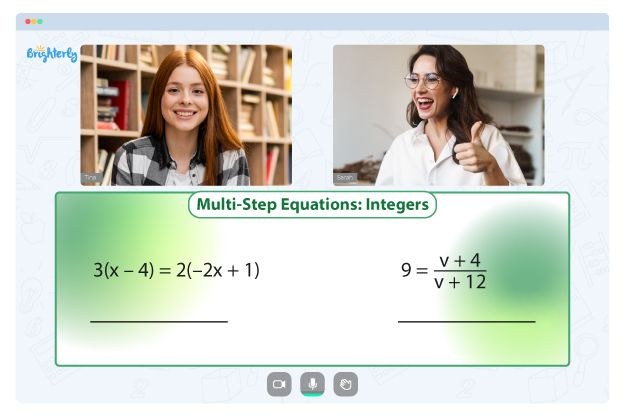# Factoring Polynomials Worksheets

The process of factoring entails division, albeit a more complex division. The factoring polynomials worksheet with answers is an excellent resource to teach your graders how to factor quadratic expressions, linear expressions, binomials, monomials, and polynomials. Below are more things you need to know about these worksheets.

## Understanding how to factor polynomials

Factoring polynomials is the opposite approach of multiplying the factors of polynomials. In a polynomial expression, all variables and constants are separated by mathematical signs like subtraction or addition.

When expressed as another linear polynomial, the zeros of polynomials are referred to as factors. After a polynomial has been factored, you will get a remainder of zero if you divide it by its factors. Also, you can factor polynomials by their GCF (Greatest Common Factor).

Factorization can be defined as the method of finding the factors of a particular mathematical expression or value. Multiplication of fractions brings an original number. For instance, 18, 9, 6, 3, and 2 are factors of 18.

Like with fractions, the factors of a polynomial are the polynomials that are multiplied to obtain the original. For instance, x² + 5x + 6 has its factors as (x + 2) (x + 3). Getting the original polynomial involves multiplying x +2 and x +3.

Factoring polynomials involves several methods:

• GCF (Greatest Common Factor)
• Difference or sum in two cubes
• Trinomials method
• General trinomials
• The difference in two squares approach

## Free factoring polynomials worksheet with answers

It is no secret that the equation aspects of mathematics are usually the most complex for students. However, finding a suitable approach to aid learning and understanding is pertinent irrespective of the problems’ difficulty. One of the most appropriate resources is factoring polynomials by grouping worksheet with answers.

Math for Kids

Is Your Child Struggling With Math?
1:1 Online Math TutoringThere are several perks of using Brighterly’s factoring polynomial worksheets. You can use them online on the Brighterly’s website. Excellent tutors design the worksheets with a perfect understanding of the concept and the ways to simplify them. When you use these learning tools, your kids will learn faster.

The polynomial factoring worksheets may not be suitable for kids below third grade. The worksheets are excellent for higher-grade kids and prepare them for subsequent phases. For instance, offering the worksheets to kids in grade five will make them more equipped and prepared for sixth grade.

The factor polynomials worksheet is designed in a stepwise way. Therefore, there is no chance of the questions overwhelming your kids. They will proceed from easy to more complex tasks, which makes understanding seamless.

## Printable factoring polynomials worksheet PDF### Factoring Polynomials Worksheets PDF

Factoring Polynomials Worksheet### Factoring Polynomials Worksheets PDF### Factoring Polynomials Worksheets PDF

Factoring Polynomials By Grouping Worksheet With Answers### Factoring Polynomials Worksheets PDF

Factoring Polynomials Worksheet PDF

The printable factoring polynomials worksheet PDF is available on the Brighterly website. You have to download and print these sheets. With the paper format, the worksheets become easier to use as you don’t have to go online whenever your kids have to study.

You can keep your kids occupied anytime when you have printable versions of worksheets. Also, since the worksheet contains answers, your kids will know when they are wrong, which enables self-learning.

### More Factoring Worksheets

Need help with Equations?• Is your child finding it challenging to grasp of equations?
• An online tutor could be the answer.

Does your child struggle with understanding of equations? Try lessons with an online tutor.